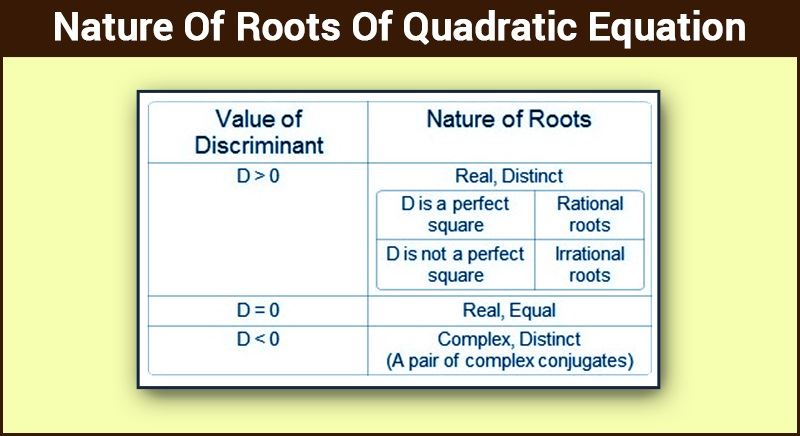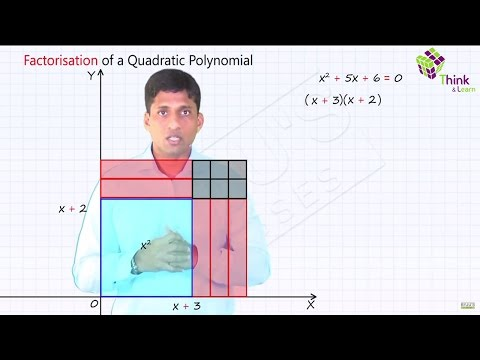Checkout JEE MAINS 2022 Question Paper Analysis : Checkout JEE MAINS 2022 Question Paper Analysis :

# Nature Of Roots Of Quadratic Equation

We already know what a quadratic equation is, let us now focus on nature of roots of quadratic equation.

A polynomial equation whose degree is 2, is known as quadratic equation. A quadratic equation in its standard form is represented as:

$$\begin{array}{l}ax^2 + bx + c\end{array}$$
=
$$\begin{array}{l}0\end{array}$$
, where
$$\begin{array}{l}a,~b ~and~ c\end{array}$$
are real numbers such that
$$\begin{array}{l}a ≠ 0\end{array}$$
and
$$\begin{array}{l}x\end{array}$$
is a variable.

The number of roots of a polynomial equation is equal to its degree. So, a quadratic equation has two roots. Some methods for finding the roots are:

• Factorization method
• Completing the square method

All the quadratic equations with real roots can be factorized. The physical significance of the roots is that at the roots of an equation, the graph of the equation intersects x-axis. The x-axis represents the real line in the Cartesian plane. This means that if the equation has unreal roots, it won’t intersect x-axis and hence it cannot be written in factorized form. Let us now go ahead and learn how to determine whether a quadratic equation will have real roots or not.

## Nature of Roots of a Quadratic Equation:Before going ahead, there is a terminology that must be understood. Consider the equation

$$\begin{array}{l}ax^2 + bx + c\end{array}$$
=
$$\begin{array}{l}0\end{array}$$

For the above equation, the roots are given by the quadratic formula as

$$\begin{array}{l}x\end{array}$$
=
$$\begin{array}{l}\frac{-b ± √({b^2 – 4ac})}{2a}\end{array}$$

Let us take a real number

$$\begin{array}{l}k > 0\end{array}$$
. Now, we know that
$$\begin{array}{l}√k\end{array}$$
is defined and is a positive quantity.

Is

$$\begin{array}{l}√{-k}\end{array}$$
a real number? The answer is no. For e.g. if we have
$$\begin{array}{l}√225\end{array}$$
, we can write it as
$$\begin{array}{l}√({15~×~15})\end{array}$$
which is equal to
$$\begin{array}{l}15\end{array}$$
.

If we have

$$\begin{array}{l}√{-225}\end{array}$$
, we can never write it as a product of two equal quantities. This is because it contains a minus sign which can only result from product of two quantities having opposite signs.

The quantity which is under square root in the expression for roots is

$$\begin{array}{l}b^2 – 4ac\end{array}$$
. This quantity is called discriminant of the quadratic equation. This is the quantity that discriminates the quadratic equations having different nature of roots. This is represented by D. So,

D =

$$\begin{array}{l}b^2 – 4ac\end{array}$$In terms of D, the roots can be written as:

$$\begin{array}{l}x\end{array}$$
=
$$\begin{array}{l}\frac{-b ± √{D}}{2a}\end{array}$$
——————————— (1)

Now, D is a real number since a, b and c are real numbers. Depending upon a, band c, the value of D can either be positive, negative or zero. Let us analyze all the possibilities and see how it affects the roots of the equation.

• D>0: When D is positive, the equation will have two real and distinct roots. This means the graph of the equation will intersect x-axis at exactly two different points.

The roots are:

$$\begin{array}{l}x\end{array}$$
=
$$\begin{array}{l}\frac{-b + √{D}}{2a} ~or~\frac{-b -√{D}}{2a}\end{array}$$
• D = 0: When D is equal to zero, the equation will have two real and equal roots. This means the graph of the equation will intersect x-axis at exactly one point. The roots can be easily determined from the equation 1 by putting D=0. The roots are:

$$\begin{array}{l}x\end{array}$$
=
$$\begin{array}{l}-\frac{b}{2a}~ or~-\frac{b}{2a}\end{array}$$

• D < 0: When D is negative, the equation will have no real roots. This means the graph of the equation will not intersect x-axis.

Let us take some examples for better understanding.

## Video Lesson

### Nature of Roots## Solved Examples

Example 1:

$$\begin{array}{l}x^2 + 5x + 6\end{array}$$
=
$$\begin{array}{l}0\end{array}$$

D =

$$\begin{array}{l}b^2 – 4ac\end{array}$$

D =

$$\begin{array}{l}5^2~-~4~×~1~×~6\end{array}$$
=
$$\begin{array}{l}25 – 24\end{array}$$
=
$$\begin{array}{l}1\end{array}$$

Since D>0, the equation will have two real and distinct roots. The roots are:

$$\begin{array}{l}x\end{array}$$
=
$$\begin{array}{l}\frac{-b + √D}{2a}\end{array}$$
or
$$\begin{array}{l}\frac{-b – √D}{2a}\end{array}$$

$$\begin{array}{l}x\end{array}$$
=
$$\begin{array}{l}\frac{-5 + √1}{2 × 1}\end{array}$$
or
$$\begin{array}{l}\frac{-5 – √1}{2~×~1}\end{array}$$

$$\begin{array}{l}x\end{array}$$
=
$$\begin{array}{l}-2\end{array}$$
or
$$\begin{array}{l}-3\end{array}$$

Example 2:

$$\begin{array}{l}x^2 + x + 1\end{array}$$
=
$$\begin{array}{l}0\end{array}$$

D =

$$\begin{array}{l}b^2 – 4ac\end{array}$$

D =

$$\begin{array}{l}1^2 – 4~×~1~×~1\end{array}$$
=
$$\begin{array}{l}1~ -~ 4\end{array}$$
=
$$\begin{array}{l}-3\end{array}$$

Since D<0, the equation will have two distinct Complex roots. The roots are:

$$\begin{array}{l}x\end{array}$$
=
$$\begin{array}{l}\frac{-b + √D}{2a}\end{array}$$
or
$$\begin{array}{l}\frac{-b – √D}{2a}\end{array}$$

$$\begin{array}{l}x\end{array}$$
=
$$\begin{array}{l}\frac{-1 + \sqrt{-3}}{2 \times 1}\end{array}$$
or
$$\begin{array}{l}\frac{-1 – \sqrt{-3}}{2 \times 1}\end{array}$$

$$\begin{array}{l}x\end{array}$$
=
$$\begin{array}{l}\frac{-1 + \sqrt{3}i}{2}\end{array}$$
or
$$\begin{array}{l}\frac{-1 – \sqrt{3}i}{2}\end{array}$$

Example 3:

$$\begin{array}{l}4x^2 + 12x + 9\end{array}$$
=
$$\begin{array}{l}0\end{array}$$

D =

$$\begin{array}{l}b^2 – 4ac\end{array}$$

D =

$$\begin{array}{l}12^2 – 4~×~4~×~9\end{array}$$
=
$$\begin{array}{l}144~-~144\end{array}$$
=
$$\begin{array}{l}0\end{array}$$

Since D = 0, the equation will have two real and equal roots. The roots are:

$$\begin{array}{l}x = +\frac{b}{2a}\end{array}$$
or
$$\begin{array}{l}-\frac{b}{2a}\end{array}$$

$$\begin{array}{l}x = +\frac{12}{2~×~4}\end{array}$$
or
$$\begin{array}{l}-\frac{12}{2~×~4}\end{array}$$

$$\begin{array}{l}x\end{array}$$
=
$$\begin{array}{l}+\frac{3}{2}\end{array}$$
or
$$\begin{array}{l}-\frac{3}{2}\end{array}$$

To solve more problems on the topic, download BYJU’S – The Learning App from Google Play Store and watch interactive videos. Also, take free tests to practice for exams.

Quiz on Nature of roots of quadratic equations